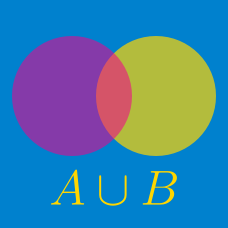Probability

# Sets - Symmetric Difference

Consider the sets \begin{aligned} A &=\{x \mid -8 < x < 4, x \text{ is an integer} \}\\ B &=\{x \mid -2 < x \leq 11, x \text{ is an integer} \}. \end{aligned} What is $\lvert A \triangle B \rvert ?$

Details and assumptions

You may choose to read the summary page Set Notation.

Consider two sets:

$A = \{ 5, 6, 7, 8, 9, 10 \} \\ B = \{ 6, 8, 10, 16, 24 \}$

What is $\lvert A \triangle B \rvert$?

Consider the sets $A=\{1,2,a+7\}, B=\{a+3, a^2, -a+10, 16\}.$ If $A \cap B=\{1, 16\},$ what is the sum of all the elements in the set $A \triangle B?$

Details and assumptions

You may choose to read the summary page Set Notation.

If sets $A$ and $B$ satisfy \begin{aligned} A & =\{23, 11, 55, 6, 4, 18, 37 \},\\ A\cap B & =\{37, 55\}, \\ \lvert A \triangle B \rvert & =5, \end{aligned} what is the sum of all the elements in the set $B?$

Details and assumptions

You may choose to read the summary page Set Notation.

If $A$ and $B$ are two sets such that $|A|=24, |B|=17, |B-A|=9,$ what is the value of $\lvert A \triangle B \rvert ?$

Details and assumptions

You may choose to read the summary page Set Notation.

×